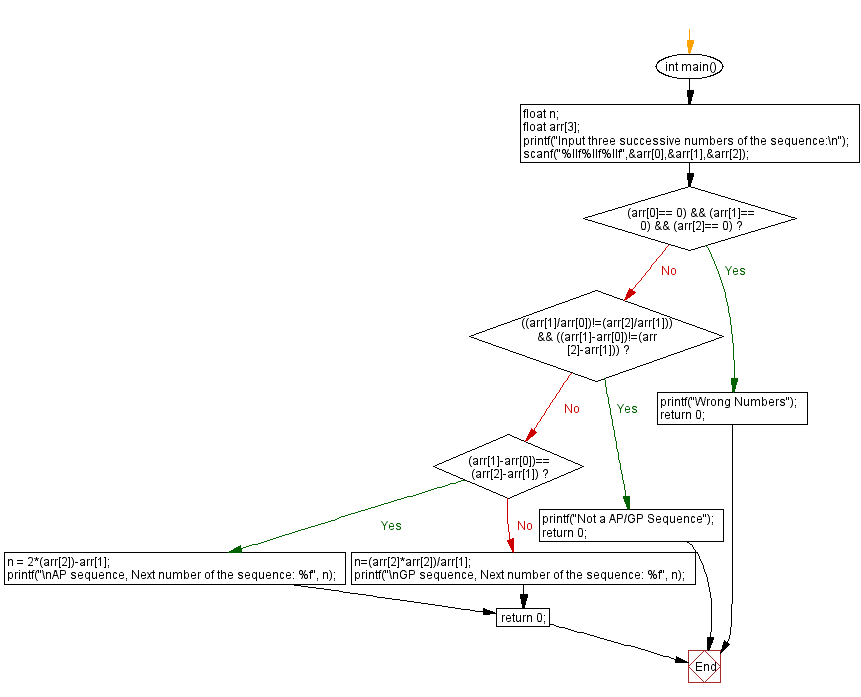﻿ C Program - Arithmetic and geometric progression series# C Exercises: Arithmetic and geometric progression series

## C Programming Challenges: Exercise-31 with Solution

Write a C program to find the type of the progression (arithmetic progression/geometric progression) and the next successive member of a given three successive members of a sequence.

According to Wikipedia, an arithmetic progression (AP) is a sequence of numbers such that the difference of any two successive members of the sequence is a constant. For instance, the sequence 3, 5, 7, 9, 11, 13,... is an arithmetic progression with common difference 2. For this problem, we will limit ourselves to arithmetic progression whose common difference is a non-zero integer. On the other hand, a geometric progression (GP) is a sequence of numbers where each term after the first is found by multiplying the previous one by a fixed non-zero number called the common ratio. For example, the sequence 2, 6, 18, 54,... is a geometric progression with common ratio 3. For this problem, we will limit ourselves to geometric progression whose common ratio is a non-zero integer.

C Code:

``````#include<stdio.h>
int main()
{
float  n;
float  arr;
printf("Input three successive numbers of the sequence:\n");
scanf("%llf%llf%llf",&arr,&arr,&arr);
if ((arr== 0) && (arr== 0) && (arr== 0))
{
printf("Wrong Numbers");
return 0;
}

if (((arr/arr)!=(arr/arr)) && ((arr-arr)!=(arr-arr)))
{
printf("Not a AP/GP Sequence");
return 0;
}

if ((arr-arr)==(arr-arr))
{
n = 2*(arr)-arr;
printf("\nAP sequence, Next number of the sequence: %f", n);
}
else
{
n=(arr*arr)/arr;
printf("\nGP sequence, Next number of the sequence: %f", n);
}
return 0;
}
``````

Sample Output:

```Input three successive numbers of the sequence:
2 6 18 54

GP sequence, Next number of the sequence: 54.000000
```

Flowchart:## C Programming Code Editor:

Contribute your code and comments through Disqus.

Previous C Programming Exercise: Prime number in strictly ascending decimal digit order.
Next C Programming Exercise: Prime number in strictly descending decimal digit order.

What is the difficulty level of this exercise?

Test your Programming skills with w3resource's quiz.

﻿